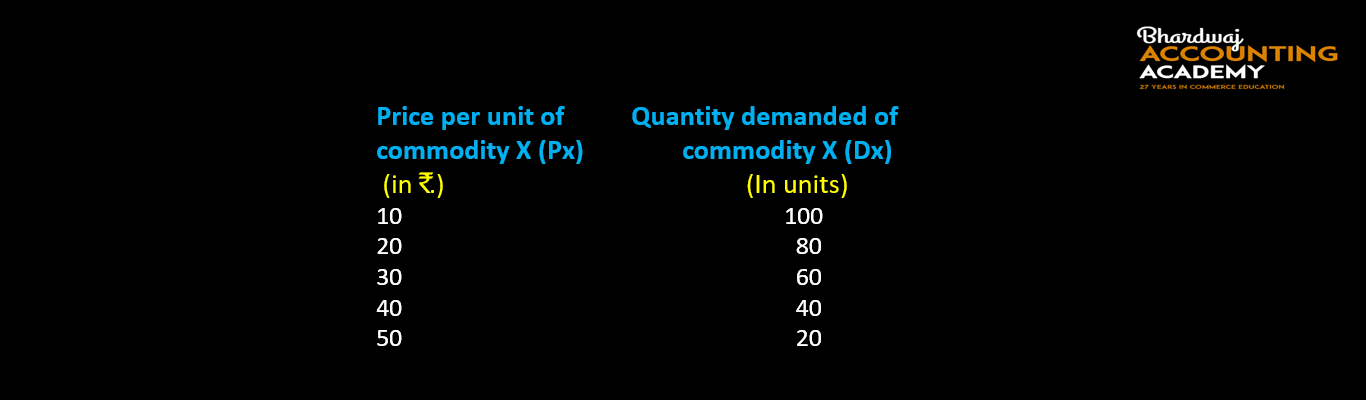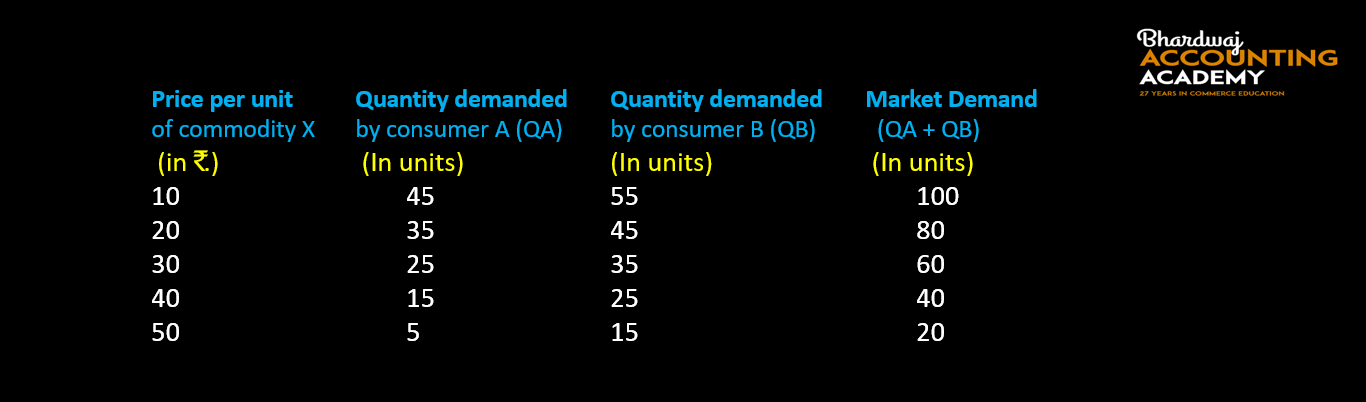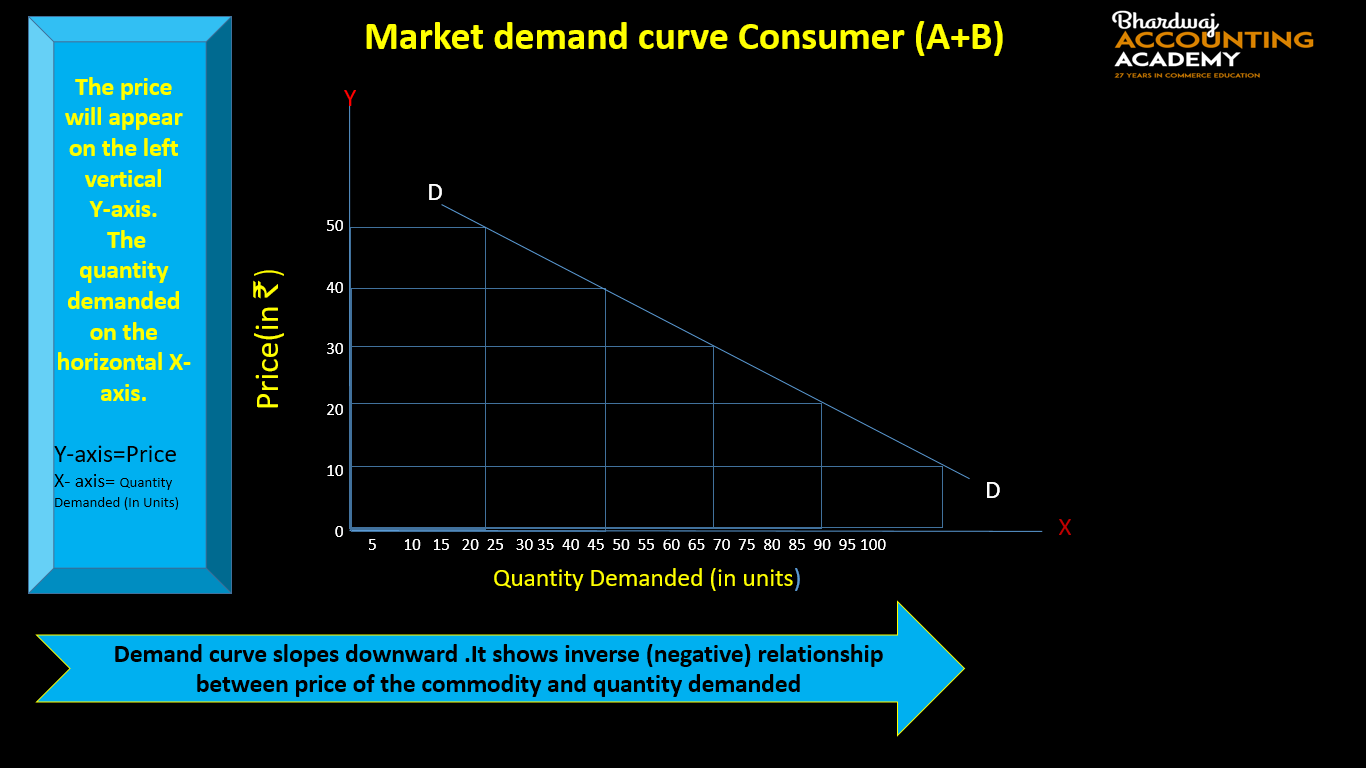# DEMAND SCHEDULE, DEMAND FUNCTION, DEMAND CURVE

## DEMAND SCHEDULE

The demand schedule is a tabular statement showing an inverse relationship between various quantities of a commodity that would be demanded and its different prices, during a given period of time.

Or

It is table showing various level of quantity demanded of a product corresponding to each given level of price.

Or

Demand schedule shows the inverse relationship between price of the commodity and its quantity demanded.

TYPES DEMAND SCHEDULE

There are two types of demand function

1. Individual demand Schedule
2. Market demand Schedule

### INDIVIDUAL DEMAND SCHEDULE

Individual demand schedule is a tabular statement showing different quantities of a commodity that an individual consumer is willing and able to buy at various levels of price, during a given period of time.

Example for individual demand schedule-### MARKET DEMAND SCHEDULE

Market demand schedule is a tabular statement showing different quantities of a commodity that all the consumers are willing and able to buy at various levels of price, during a given period of time.

Example for Market demand schedule-ALSO READ : SUPPLY AND ITS DETERMINANTS/FACTORS AFFECTING SUPPLY

# DEMAND

## DEMAND FUNCTION

Demand Function shows the functional relationship (cause and effect relationship) between the demand for a product or goods and factors affecting demand.

Or

Demand function is an algebraic expression that shows the functional relationship between the demand for a commodity and its various determinants(Factors) affecting demand.

or

A demand function shows the functional relationship between the quantity demanded and the factors on which demand depends on.

TYPES DEMAND FUNCTION

There are two types of demand function

1. Individual demand Function
2. Market demand Function

### INDIVIDUAL DEMAND FUNCTION

Individual demand function refers to the functional relationship between individual demand and the factors affecting individual demand. It is expressed as:

Dx = f (Px, Pr, Y, T, F…)

Where,

Dx = Demand for Commodity x;

Px = Price of the given Commodity x;

Pr = Prices of Related Goods;

Y = Income of the Consumer;

T = Tastes and Preferences;

F = Expectation of Change in Price in future.

### MARKET DEMAND FUNCTION

Market demand function refers to the functional relationship between market demand and the factors affecting market demand. Market demand function can be expressed as:

Dx = f(Px, Pr, Y, T, F, PO, S, D, G….)

Where,

Dx = Market demand of commodity x;

Px = Price of given commodity x;

Pr = Prices of Related Goods;

Y = Income of the consumers;

T = Tastes and Preferences;

F = Expectation of Change in Price in future;

P0 = Size and Composition of population;

S = Season and Weather;

D = Distribution of Income.

G = Government policy.

## DEMAND CURVE

Demand curve is a graphical representation of demand schedule, express the relationship between different quantities of a commodity at different possible prices .

Or

Demand curve is a graphical representation of demand schedule, it shows the inverse relationship between the price of a commodity and its quantity demanded during a given period of time.

TYPES DEMAND CURVE

There are two types of demand curve

1. Individual demand curve
2. Market demand curve

### INDIVIDUAL DEMAND CURVE

Individual Demand curve refers to a graphical representation of individual demand schedule.

Or

Individual Demand curve is a curve showing different quantities of a commodity that a consumer is willing and able to buy at various levels of price, during a given period of time.# DEMAND

### MARKET DEMAND CURVE

Market Demand curve refers to a graphical representation of Market demand schedule.

Or

Market Demand curve is a curve showing different quantities of a commodity that all the consumers are willing and able to buy at various levels of price, during a given period of time.• Individual demand refers to quantity of a commodity that a consumer is willing and able to buy, at each possible price during a given period of time.
• Market demand refers to the quantity of a commodity that all consumers are willing and able to buy, at each possible price during a given period of time.

### 1 thought on “Demand Schedule, Demand Function, Demand Curve”

1.Thank You Sir